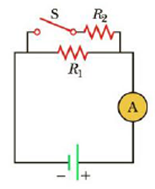Chapter 18.3, Problem 18.6QQ

Chapter
Section
Textbook Problem

When the switch is open in Figure 18.8, power Po is delivered to the resistor R1. When the switch is closed, which of the following is true about the power Pc delivered to R1? (Neglect the internal resistance of the battery.) (a) Pc < Po (b) Pc = Po (c) Pc > PoFigure 18.8 (Quick Quizzes 18.5 and 18.6)

To determine
The power delivered to R1 when the switch is open.

Explanation

Given Info: The power delivered to the resistor R1 when the switch is closed is Pc . The power delivered to the resistor R1 when the switch is open is Po .

Explanation:

The expression of power is,

Po=V2R1

• Po is the power dissipated by the resistor when the switch is open,
• V is the potential across the resistor,
• R1 is the resistance of the resistor 1,

When the switch is closed, resistor R1 and R2 are in parallel fashion with the power supply.

Conclusion:

When the switch is open the potential across the Resistor R1 is the potential across the power supply

Still sussing out bartleby?

Check out a sample textbook solution.

See a sample solution

The Solution to Your Study Problems

Bartleby provides explanations to thousands of textbook problems written by our experts, many with advanced degrees!

Get Started